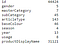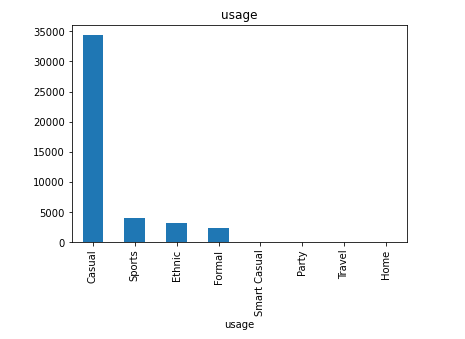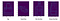# Introduction:

The magic of machine learning is the more we understand the concepts and the idea of origination, the easier it becomes for us. Here in this article we will look into the approach of using Histogram of Oriented Gradients in image classification and image recommendation. The data can be found here and the Jupyter Notebook containing the solution can be found here.

# The Dataset:Source: Kaggle Fashion Image Classification Dataset (Small)Unique values for each column. For each of gender, masterCategory, subCategory, gender, usage and season columns KNN Classifiers have been used for image classification followed by, K Nearest Neighbours being used for image recommendationNumber of records for different classes (Only Top 10 were shown) under each column

# Steps for Computing HOG:

HOG is a technique for transforming an image to a histogram of gradients and later use the histograms to make a 1D matrix which would be used for training a model.

`import osimport numpy as npimport pandas as pdimport cv2 as cvfrom pathlib import Pathimport warningsfrom skimage.feature import hogimport tqdmfrom sklearn.neighbors import KNeighborsClassifierfrom sklearn import metricsfrom sklearn.model_selection import train_test_splitfrom sklearn.preprocessing import MinMaxScalerfrom sklearn.neighbors import NearestNeighborswarnings.filterwarnings("ignore")pd.options.display.max_columns = None`
`all_images = []#labels = []def load_image(ids,path=image_folder):    img = cv.imread(image_folder+ids+'.jpg',cv.IMREAD_GRAYSCALE) #load at gray scale    #img = cv.cvtColor(img, cv.COLOR_BGR2GRAY) #convert to gray scale    return img,ids#20k samples were taken for modelingfor ids in tqdm(list(styles.id)[:20000]):    img,ids = load_image(str(ids))    if img is not None:        all_images.append([img,int(ids)])    #labels.append(ids)len(all_images)`
`def resize_image(img,ids):    return cv.resize(img, (60, 80),interpolation =cv.INTER_LINEAR)    all_images_resized = [[resize_image(x,y),y] for x,y in all_images]len(all_images_resized)`
`##HOG Descriptor#Returns a 1D vector for an imageppcr = 8ppcc = 8hog_images = []hog_features = []for image in tqdm(train_images): blur = cv.GaussianBlur(image,(5,5),0) #Gaussian Filtering fd,hog_image = hog(blur, orientations=8, pixels_per_cell=(ppcr,ppcc),cells_per_block=(2,2),block_norm= ‘L2’,visualize=True) hog_images.append(hog_image) hog_features.append(fd)hog_features = np.array(hog_features)hog_features.shape`
`#normalization by 'L2-Hys'out = block / np.sqrt(np.sum(block ** 2) + eps ** 2)        out = np.minimum(out, 0.2)        out = out / np.sqrt(np.sum(out ** 2) + eps ** 2)`The idea of using gradient direction in modeling is because human cortex system works in similar way. Cerebral Cortex gets attention when human sees some objects into a particular direction, or human changes the angle with the object in order to see it better
`X_train, X_test, y_train, y_test = train_test_split(hog_features,df_labels['class'],test_size=0.2,stratify=df_labels['class'])print('Training data and target sizes: \n{}, {}'.format(X_train.shape,y_train.shape))print('Test data and target sizes: \n{}, {}'.format(X_test.shape,y_test.shape))=============================================                   Training data and target sizes: (15998, 1728), (15998,)Test data and target sizes: (4000, 1728), (4000,)`
`test_accuracy = []scaler = StandardScaler()X_scaled = scaler.fit_transform(X_train)classifier = KNeighborsClassifier(n_neighbors=3,algorithm='brute')classifier.fit(X_scaled, y_train)test_accuracy = classifier.score(scaler.transform(X_test), y_test)print(test_accuracy)`
`list_of_categories = categories +['Others']print("Classification Report: \n Target: %s \n Labels: %s \n Classifier: %s:\n%s\n"      % (target,list_of_categories,classifier, metrics.classification_report(y_test, y_pred)))df_report = pd.DataFrame(metrics.confusion_matrix(y_test, y_pred),columns = list_of_categories )df_report.index = [list_of_categories]df_report`
`#test image with idtest_data_location = root+'/test/'img = cv.imread(test_data_location+'1570.jpg',cv.IMREAD_GRAYSCALE) #load at gray scaleimage = cv.resize(img, (60, 80),interpolation =cv.INTER_LINEAR)ppcr = 8ppcc = 8hog_images_test = []hog_features_test = []blur = cv.GaussianBlur(image,(5,5),0)fd_test,hog_img = hog(blur, orientations=8, pixels_per_cell=(ppcr,ppcc),cells_per_block=(2,2),block_norm= 'L2',visualize=True)hog_images_test.append(hog_img)hog_features_test.append(fd)hog_features_test = np.array(hog_features_test)y_pred_user = classifier.predict(scaler.transform(hog_features_test))#print(plt.imshow(hog_images_test))print(y_pred_user)print("Predicted MaterCategory: ", mapper[mapper['class']==int(y_pred_user)]['masterCategory'])`
`scaler_global = MinMaxScaler()final_features_scaled = scaler_global.fit_transform(hog_features)    neighbors = NearestNeighbors(n_neighbors=20, algorithm='brute')neighbors.fit(final_features_scaled)distance,potential = neighbors.kneighbors(scaler_global.transform(hog_features_test))print("Potential Neighbors Found!")neighbors = []for i in potential:    neighbors.append(i)recommendation_list = list(df_labels.iloc[neighbors]['id'])recommendation_list`

# Conclusion:

The above explanation shows what is the intuition behind HOG, how we can use it to describe features of an image. In the next, the HOG features were computed and used in a KNN classifier and later in finding out K Nearest Neighbors. Both the cases achieve high level of accuracy without using any deep learning methods. There was a few cases where the image was mislabelled or image having multiple objects but labelled in a single class which affected our model. Next step would be to identify the root cause of misclassification and making a better classification and recommendation engine.

## The Startup

Get smarter at building your thing. Join The Startup’s +724K followers.

## The Startup

Get smarter at building your thing. Follow to join The Startup’s +8 million monthly readers & +724K followers.## The Startup

Get smarter at building your thing. Follow to join The Startup’s +8 million monthly readers & +724K followers.

## More From Medium

Medium is an open platform where 170 million readers come to find insightful and dynamic thinking. Here, expert and undiscovered voices alike dive into the heart of any topic and bring new ideas to the surface. Learn more

Follow the writers, publications, and topics that matter to you, and you’ll see them on your homepage and in your inbox. Explore

If you have a story to tell, knowledge to share, or a perspective to offer — welcome home. It’s easy and free to post your thinking on any topic. Write on Medium